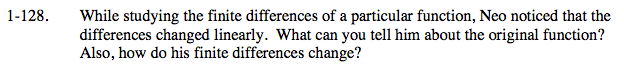### Home > CALC > Chapter 1 > Lesson 1.3.3 > Problem1-128

1-128.A linear changing difference is a difference that increases or decreases in greater amounts each time. For example, if f(1) = 1, f(2) = 4, f(3) = 9, f(4) = 16, then the differences are 3, 5 and 7, respectively, and changing linearly, in this case with the linear rule, 2x + 1. What kind of function has this kind of change?